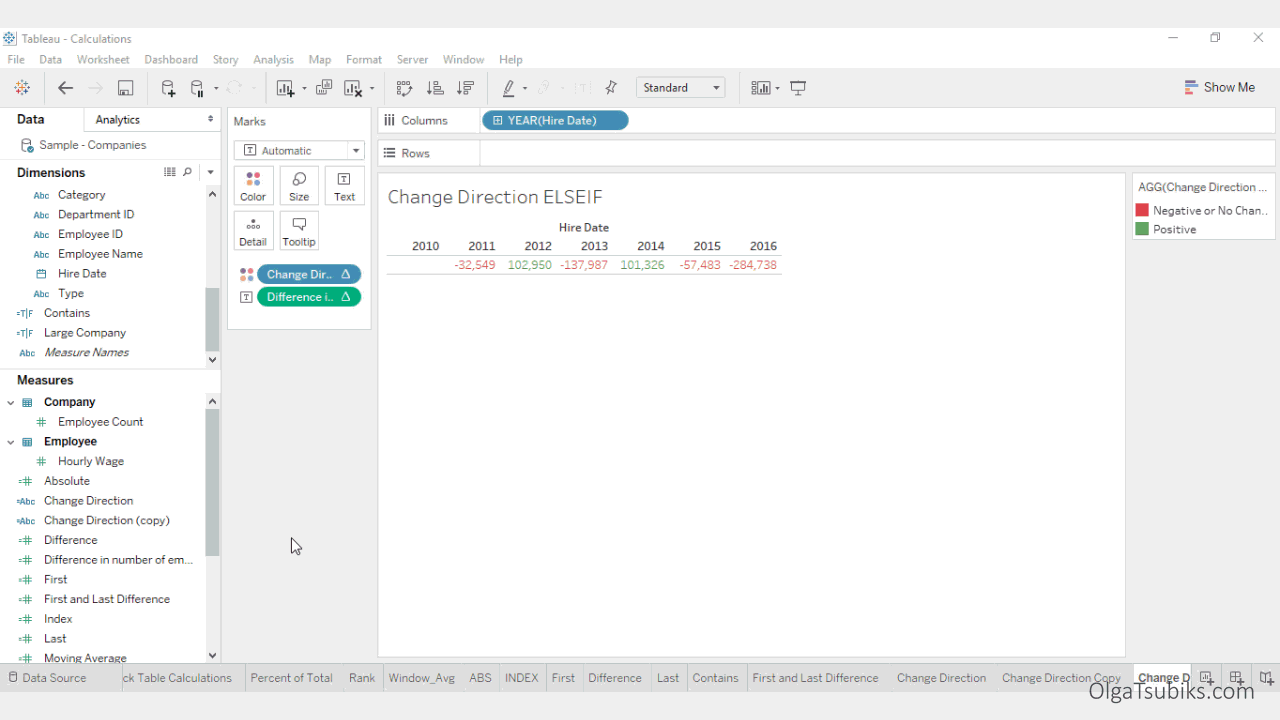PART VII

## IIF(test, then, else, [unknown])

Use the IIF function to perform logic tests and return appropriate values. The first argument, or test, must be a Boolean: either a Boolean field in the data source, or the result of a logical expression using operators (or a logical comparison of AND, OR, or NOT). If the test evaluates to TRUE, then IIF returns the THEN value. If the test evaluates to FALSE, then IIF returns the ELSE value.

A Boolean comparison may also yield the value UNKNOWN (neither TRUE nor FALSE), usually due to the presence of null values in the test. The final argument to IIF is returned in the event of an UNKNOWN result for the comparison. If this argument is left out, Null is returned.

For example, let's use our difference in number of employees calculation, to find out if the difference is positive, negative or unknown.Solution: Create a new calculation called Change Direction. Add the following logic statement: IIF([Difference in number of employees]>0,"Positive","Negative or No Change","Unknown"). Add the new calculation on the Color Marks Card.

## IF test THEN value END / IF test THEN value ELSE else END

This function is similar to the IIF() function. The IIF statement distinguishes TRUE, FALSE and UNKNOWN; whereas an IF statement only worries about TRUE and not true (which includes both FALSE and UNKNOWN). Use the IF THEN ELSE function to perform logic tests and return appropriate values. The IF THEN ELSE function evaluates a sequence of test conditions and returns the value for the first condition that is true. If no condition is true, the ELSE value is returned. Each test must be a Boolean: either a Boolean field in the data source, or the result of a logical expression. The final ELSE is optional, but if it is not provided and there is no true test expression, then the function returns Null. All of the value expressions must be of the same type.

For example, let's edit our Change Direction calculation to use this function instead of IIF().Solution:

Right-click on the Change Direction calculation and select Edit. Replace it with the following calculation: IF [Difference in number of employees]>0 THEN "Positive" ELSE "Negative or No Change" END.

## IF test1 THEN value1 ELSEIF test2 THEN value2 ELSE else END

Use this version of the IF function to perform logic tests recursively. There is no built-in limit to the number of ELSEIF values you can use with an IF function in Tableau.

For example, let's edit our Change Direction calculation to use this function.Solution: Right-click on the Change Direction calculation and select Edit. Replace it with the following calculation: IF [Difference in number of employees]>0 THEN "Positive" ELSEIF [Difference in number of employees]<0 THEN "Negative" ELSE "Neutral" END.